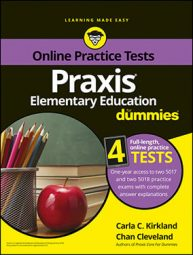##### Praxis Elementary Education For Dummies with Online Practice TestsThe Math and Science sections of the Praxis Elementary Education exam contain questions where you will need to be familiar with different forms of measurement.

Measurements can be described in various types of units. English system units for distance include inches, feet, yards, and miles. They are based on each other. A foot is 12 inches, a yard is three feet, and a mile is 5,280 feet. Area measurements are given in those units squared, or to the second power (having an exponent of 2), and volume can be given in those units cubed (to the third power) or in another subsystem of English units based on the cup. A cup is about the size of a small drink that goes in the type of object called a cup, a pint is two cups, a quart is two pints, and a gallon is four quarts.

The metric system's basic unit for distance is the meter, and other distance units are based on the meter. For example, a centimeter is one-hundredth of a meter, and a kilometer is 1,000 meters. Those are one-dimensional measurements. Two-dimensional units are the squares of one-dimensional units. For example, a rectangle with a length of 5 centimeters (cm) and a width of 2 cm would have an area of 10 cm2. Volume often involves the same kinds of distance units with an exponent of 3 because volume is three-dimensional. However, the metric system also uses the liter for measures of volume. One liter is equal to 1,000 cm3. The table lists metric prefixes.

## Practice question

1. 285.496 decameters is equal to how many centimeters? A. 0.285496 B. 28.5496 C. 28,549.6 D. 285,496

## Answer and explanation

1. The correct answer is Choice (D). A decameter is 10 meters, a meter is 10 decimeters, and a decimeter is equal to 10 centimeters. Therefore, a decameter is

or 1,000 centimeters. Any number of decameters is the same as 1,000 times that many centimeters. Thus, 285.496 decameters is 285,496 centimeters. To multiply a decimal number by 1,000, you can simply move the decimal three places to the right. For every 10 you multiply a decimal number by, move the decimal one place to the right. Also, you can make a list of the metric prefixes in order. Every space you move down from bigger units to smaller units requires that you multiply by 10 to compensate. If you move down three prefixes, as you do in this case, you multiply the number by

The other choices involve moving the decimal incorrectly.